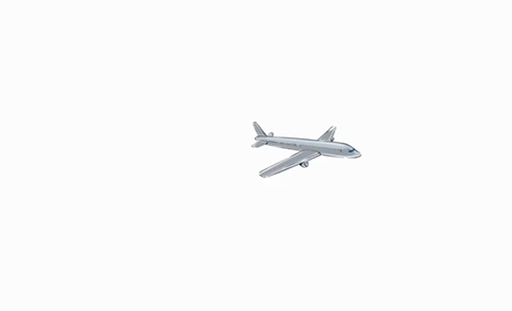An introduction to geology

Start this free course now. Just create an account and sign in. Enrol and complete the course for a free statement of participation or digital badge if available.

Free course

# 2.5 From soil to soda cans

An aluminium drinks can is made of about 15 g of aluminium. Aluminium is the third most abundant element in the Earth’s crust (the bit we can ‘get at’) after oxygen and silicon, but is only about 8.4% of the material. If you wanted to extract the necessary aluminium from the Earth's crust, you would need to grind up 178 g of material to get enough aluminium to make one can:

How about a fairly typical rock, like a granite?

Well, granite is ~ 14% alumina (Al2O3). First you need to convert this to % aluminium, which we do by multiplying the percentage of Al2O3 by the percentage of Al in Al2O3:

The atomic mass of Al is 27. The atomic mass of O is 16.

So you’d actually need more granite to get the necessary aluminium than if you just ground up the crust.

Don't worry if this seems a bit too complicated – this video clip reiterates the above material and explores the maths a bit more closely.Skip transcript: Video 2.2 Extracting enough aluminium

#### Transcript: Video 2.2 Extracting enough aluminium

SPEAKER:
How much material does it take to make a soda can? An aluminium drinks can is made of about 15 grams of aluminium. Aluminium is one of the most abundant elements in the Earth’s crust. This is the bit that we can actually get at. Aluminium is the third-most abundant element after oxygen and silicon, but it’s still only 8.4% of the material.
If we were to remove all the crust from the Earth, grind it up, and extract the aluminium, you would need – 15 grams of aluminium divided by 8.4% over 100 equals 178 grams of material to make one can. In this scenario, you would also need to deal with the fact that the world would no longer have a crust. Oh, well.
Now, how about a fairly typical rock like a granite? Well, granite is about 14% alumina. Alumina is Al2O3. First, you need to convert this to a percentage, aluminium, which we do by multiplying the percentage of alumina by the percentage of aluminium in alumina. To do this, we need to use the atomic masses of aluminium, which is 27, and oxygen, which is 16. The atomic masses are just the mass of each molecule of each element in a handy unit, the atomic mass unit, which makes the numbers easy to handle. So going through the maths, aluminium atomic mass is 27. Oxygen atomic mass is 16.
In alumina, from the chemical formula, we know that there are two molecules of aluminium and three of oxygen, so we can work out the percentage of alumina which is aluminium. That works out as 53%, and use that to calculate that granite is 7.4% aluminium. So let’s look at that maths in detail. You have 2 times aluminium plus 3 times oxygen in alumina, and that gives you 102. 2 times aluminium is 54, so 14% of Al2O3 times 54 over 102 gives us the answer that granite is 7 and 7/17% aluminium. That’s 7.4% aluminium. If we expand that out and look at the mass, 15 grams of aluminium over 7 and 7/17 divided by 100 gives you 202 and 8/21 of granite.
So that all figures out that to make a soda can from granite alone, you need 202.4 grams of granite, so you’d actually need more granite than if you just ground up the whole crust.
End transcript: Video 2.2 Extracting enough aluminium
Video 2.2 Extracting enough aluminium
Interactive feature not available in single page view (see it in standard view).

What about bauxite? Bauxite is formed from rocks like granite – how effective is the geological process in concentrating the aluminium? Test your understanding with the next activity.

OUFL_1009